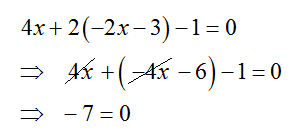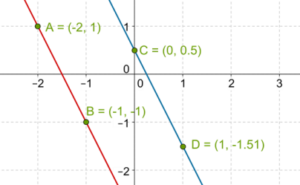# Substitution Method

Go back to  'Pair of Linear Equations in Two Variables'

We have seen how to graphically determine the solution to a pair of linear equations. However, this technique is generally not convenient in every case, especially if the answer (the coordinates of the point of intersection) is complicated. For example, suppose that two lines intersect in a point whose coordinates are given by:

$\left( {\frac{3}{{256}},\; - \frac{{27}}{{193}}} \right)$

If we were to plot the two lines graphically, it would be very difficult to accurately determine this answer (try plotting this point on a graph, and ask yourself, how accurate can I be?). At best, we could have found out the point of intersection approximately. It should be clear that if we have to find out the exact solution to a pair of linear equations, we must use algebraic methods.

The first algebraic method we will discuss is substitution. The idea is to use one of the pair of equations to express one unknown quantity in terms of the other, and then substituting this expression into the second equation, so that we are left with an equation with only one unknown, which can easily be solved to find that unknown. And once we have any one of the two unknowns, the other can be found out easily. Let’s discuss this through an example. Consider the following pair of linear equations:

$\begin{array}{l}x + 2y - 7 = 0\;\;\;\; & ...(1)\\2x - 5y + 13 = 0\;\;\;\; & ...(2)\end{array}$Let’s rearrange the first equation to express y in terms of x, as follows:$\begin{array}{l}x + 2y - 7 = 0\\ \Rightarrow \;\;\;2y = 7 - x\\ \Rightarrow \;\;\;y = \frac{{7 - x}}{2}\end{array}$

This expression for y can now be substituted in the second equation, so that we will be left with an equation in x alone:

\begin{align}&2x - 5y + 13 = 0\\ &\Rightarrow \;\;\;2x - 5\left( {\frac{{7 - x}}{2}} \right) + 13 = 0\\ &\Rightarrow \;\;\;2x - \frac{{35}}{2} = \frac{{5x}}{2} + 13 = 0\\ &\Rightarrow \;\;\;2x + \frac{{5x}}{2} = \frac{{35}}{2} - 13\\ &\Rightarrow \;\;\;\frac{{9x}}{2} = \frac{9}{2}\\ &\Rightarrow \;\;\;x = 1\end{align}

Once we have the value of x, we can plug this back into any of the two equations to find out y. Lets plug it into the first equation:

$\begin{array}{l}x + 2y - 7 = 0\\ \Rightarrow \;\;\;(1) + 2y - 7 = 0\\ \Rightarrow \;\;\;2y = 7 - 1 = 6\\ \Rightarrow \;\;\;y = 3\end{array}$

The final solution is:

$x = 1,\;y = 3$

It should be clear why this process is called substitution. We express one variable in terms of another using one of the pair of equations, and substitute that expression into the second equation.

Example 1: Solve the following pair of linear equations by substitution:

$\begin{array}{l}2x + 3y = 8 & & ...(1)\\3x + y + 2 = 0 & & ...(2)\end{array}$

Solution: Using the first equation, we express x in terms of y:

$\begin{array}{l}2x = 8 - 3y\\ \Rightarrow \;\;\;x = \frac{{8 - 3y}}{2}\end{array}$

Now, we substitute this expression into the second equation to obtain an equation which contains only one variable, that is, y:

$\begin{array}{l}3x + y + 2 = 0\\ \Rightarrow \;\;\;3\left( {\frac{{8 - 3y}}{2}} \right) + y + 2 = 0\\ \Rightarrow \;\;\;12 - \frac{{9y}}{2} + y + 2 = 0\\ \Rightarrow \;\;\;\frac{{7y}}{2} = 14\\ \Rightarrow \;\;\;y = 4\end{array}$

Once we have evaluated the y part of the solution, we plug this back into any one of the two equations to obtain the x value of the solution. Let’s plug the y value into the second equation:

$\begin{array}{l}3x + y + 2 = 0\\ \Rightarrow \;\;\;3x + \left( 4 \right) + 2 = 0\\ \Rightarrow \;\;\;3x = - 6\\ \Rightarrow \;\;\;x = - 2\end{array}$

Thus, the solution is:

$x = - 2,\;y = 4$

Example 2: Solve the following pair of linear equations by substitution:

$\begin{array}{l}2x + y + 3 = 0 & & ...(1)\\4x + 2y - 1 = 0 & & ...(2)\end{array}$

Solution: Using the first equation, we express y in terms of x:

$y = - 2x - 3$

Now, we substitute this into the second equation:What does this mean? How can $$- 7$$ be equal to 0? This is clearly an impossible equality. Why this is happening is because the pair of linear equations is inconsistent, as the following graph shows (two points have been plotted on each line):The fact that we are getting an inconsistent equality upon substitution is thus explained by the fact that this pair of equations is inconsistent. No solution exists to this pair!

Example 3: Solve the following pair of linear equations by substitution:

$\begin{array}{l}x - 2y + 1 = 0 & & ...(1)\\3x - 6y = - 3 & & ...(2)\end{array}$

Solution: Using the first equation, we express y in terms of x:

$\begin{array}{l}x - 2y + 1 = 0\\ \Rightarrow \;\;\;2y = x + 1\\ \Rightarrow \;\;\;y = \frac{{x + 1}}{2}\end{array}$

Now, we substitute this into the second equation:

$\begin{array}{l}3x - 6\left( {\frac{{x + 1}}{2}} \right) = - 3\\ \Rightarrow \;\;\;3x - 3\left( {x + 1} \right) + 3 = 0\\ \Rightarrow \;\;\; - 3 + 3 = 0\\ \Rightarrow \;\;\;0 = 0\end{array}$

Once again, we have something unexpected. An equality with no variables, and an equality which is true regardless of x and y. This points to the fact that the system has infinitely many solutions, that is, it is a consistent system but the two linear equations happen to be identical:

$\begin{array}{l}{\rm{Equation}}\;2:\\3x - 6y = - 3\\ \Rightarrow \;\;\;3x - 6y + 3 = 0\\ \Rightarrow \;\;\;3\left( {x - 2y + 1} \right) = 0\\ \Rightarrow \;\;\;x - 2y + 1 = 0\\\;\;\;\;\;\;[{\rm{same}}\;{\rm{as}}\;(1)]\end{array}$

To summarize: suppose that we carry out the substitution as our method tells us. Three cases are possible:

1. We get a well-defined equality in one variable. This means that the pair of equations will have a unique solution.

2. We get an impossible equality, which means that the pair is inconsistent, and hence no solutions exist.

3. We get an equality which is actually an identity, that is, it is universally true. This means that the pair of equations has infinitely many solutions.

Pair of Linear Equations in 2 Variables
Pair of Linear Equations in 2 Variables
grade 10 | Questions Set 2
Pair of Linear Equations in 2 Variables
Pair of Linear Equations in 2 Variables
grade 10 | Questions Set 1